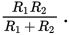# SAT Math Multiple Choice Question 450: Answer and Explanation

### Test Information

Question: 450

15.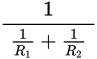In electronic circuits, resistors are often paired to manage the flow of the electrical current. To find the total resistance of a pair of parallel resistors, electricians use the formula shown above, where R1 is the resistance of the first resistor and R2 is the resistance of the second resistor. Which of the following is another way to represent this formula?

• A.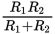• B.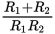• C.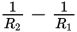• D.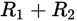Explanation:

A

Difficulty: Hard

Category: Passport to Advanced Math / Exponents

Strategic Advice: Simplifying a complex rational expression requires planning and patience. Here, you need to write the denominator of the big expression as a single fraction, and then you can simply "flip it" to adjust for the "1 over."

Getting to the Answer: Start by writing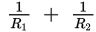as a single term. To do this, find the common denominator and write each piece of the sum in terms of that denominator. The common denominator is R1R2.But remember, this fraction is the denominator under 1, so you need to write the reciprocal (flip it); the correct expression is